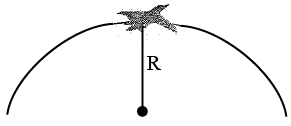# ISRO Scientist or Engineer Mechanical 2011

Instructions

For the following questions answer them individually

Question 21

# If two objects are weighed in water and both of them lose the same weight, then the two objects must have identicalQuestion 22

# 1kg of moist air of RH 70% at $$21^\circ C$$ is cooled at constant pressure of 1 bar to $$5^\circ C.$$ The vapour pressure at $$21^\circ C$$ and $$5^\circ C$$ are 0.025 bar and 0.0087 bar. The percentage of water vapour that condenses into water, at $$5^\circ C$$ isQuestion 23

# Two spherical balis of same material and surface finish have their diameters in the ratio of 2:1. Both are heated to same temperature and allowed to cool by radiation. Rate of cooling of big ball as compared to smaller one will be in the ratio ofQuestion 24

# Ninety kilograms of ice at $$0^\circ C$$ are completely melted. Find the entropy change, in kJ/K, if $$T_2 = 0^\circ C.$$ (Latent heat of fusion is 318.5 kJ/kg.K)Question 25

# A solid shaft of 100mm diameter transmits 160 HP at 200rpm. The modulus of rigidity $$c = 8 \times 10^5 kg/cm^2$$ .Then the maximum angle of twist for a length of 6 meterisQuestion 26

# A perfect gas at $$27^\circ C$$ is heated at constant pressure till its volume is double. The final temperature isQuestion 27

# The stagnation temperature of an isentropic flow of air (k = 1.4) is 360 K. If the temperature is 200 K at a section, then the Mach numberof the flow will beQuestion 28

# Air at $$20^\circ C$$ blows over a plate of $$50 cm \times 75 cm$$ maintained at $$250^\circ C.$$ If the convection heat transfer coefficient is $$25W/m^{2^\circ} C$$ , the heat transfer rate isQuestion 29

# A small plastic boat loaded with pieces of steel rods is floating in a bath tub. If the cargo is dumped into the water allowing the boatto float empty, the water level in the tub willQuestion 30

# When a jet plane flying at 300 m/s is at the very top of its trajectory, the apparent weight of a passenger is one half of her actual weight. Find the radius of curvature R of the flight path at this point. Use $$g = 10 m/s^2.$$OR